#Units of Conversion: Cubic (Volume) MeasureBasic to the understanding of unit conversion is the understanding of equivalence, the understanding of the multiplicative identity of 1, and how the two are related. For a review of these concepts, click on Review.

You will also need to multiply and reduce fractions. If you have forgotten how to manipulate fractions, visit Fractions for Adults on SOSMath for an in-depth review.

Review the following table of Standard Units of Conversion to see if you can create fractions that have a value of 1. You can click to examples following each table.

Cubic Measure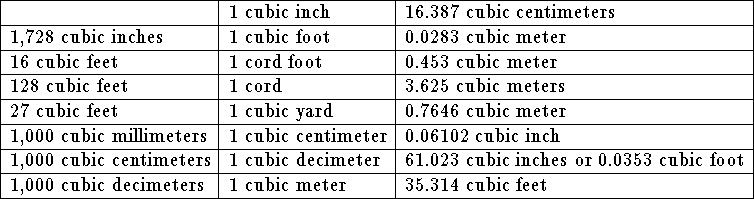Example 1:What exactly does 1 cubic foot mean?

One cubic foot means 1 foot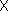1 foot1 foot or 1 foot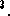Example 2:Convert 50 cubic yards to cubic feet.

Solution:From the above table you can see a link between cubic feet and cubic yards.

• 50 cubic yards can be written as 50 x 1 cubic yard.
• Since 27 cubic feet is equivalent to 1 cubic yard, the fraction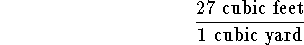is equivalent to 1.

• Multiply 50 x 1 cubic yard by 1 in the equivalent form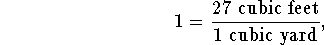to obtain 50 x 1 cubic yard x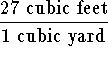.

• Rewrite the above expression as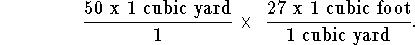• Multiply the two fractions: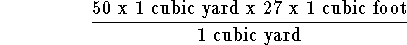• Rearrange the numerator: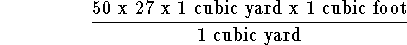• Write the above fraction as a product of fractions so that some of the fractions have a value of 1: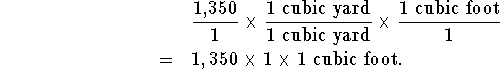• The answer is 1,350 cubic feet, or 1,350 feet3.
A longer way to work the same problem is as follows.
• 1 cubic yard can be written as 1 yard x 1 yard x 1 yard.
• 50 cubic yards can be written 50 x 1 yard x 1 yard x 1 yard.
• Note that 1 yard can be written as 1 yard x 1 and the 1 can be written as a fraction where the numerator and the denominator are equivalent.
• Write 1 yard x 1 as 1 yard x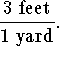• This can be further simplified to read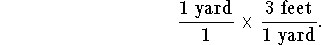•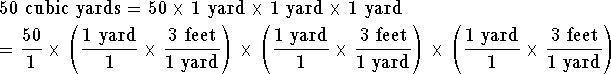• Rearrange the fractions within the parenthesis.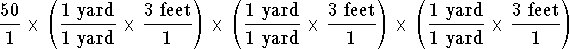• Simplify.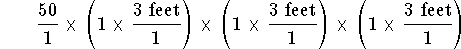• Simplify more.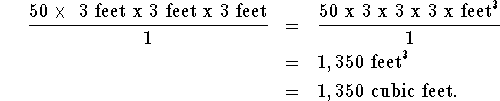• The answer is 1,350 cubic feet.

If you would like to review another example, click on Example.

If you would like to work some problems click on Problems.[Units of Conversion]S.O.S MATHematics home page

Do you need more help? Please post your question on our S.O.S. Mathematics CyberBoard.Author: Nancy Marcus